﻿ InnovateFPGA | Americas | EM030 - Neural cryptography system based on TPM-networks
Annual: 2019

📁Digital Design
👤Viktoriya Kosolapova
(National Research University Higher School of Economics)
📅Oct 17, 2019👀 2712   💬 4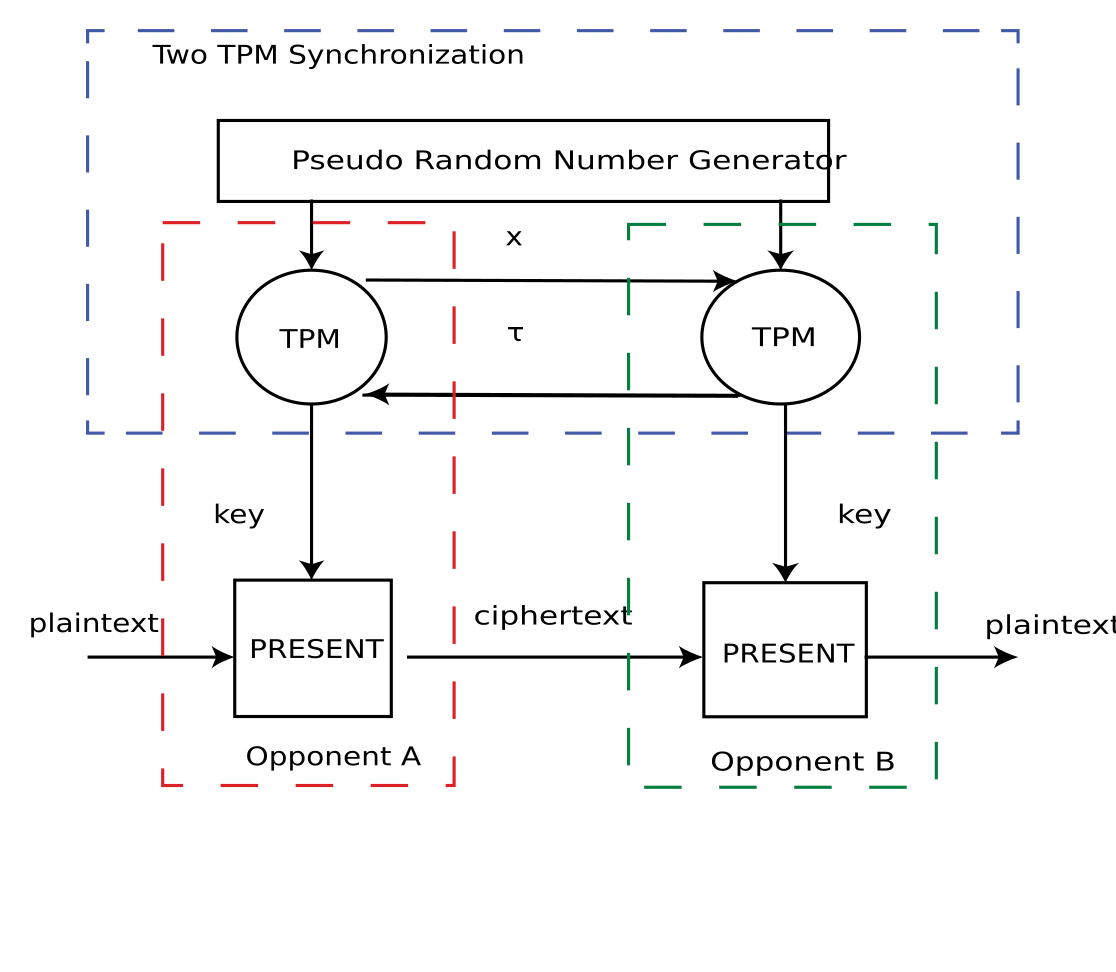EM030 » Neural cryptography system based on TPM-networks

### Description

This project is aimed at the development of hardware cryptosystem based on TPM (Tree Parity Machine). TPM is a particular multi-layer feedforward neural network structure employing the mutual learning concept for neural cryptography. Two TPMs synchronization is used as a secret key exchange protocol. Sent information is encrypted with PRESENT block cipher. FPGA implementation advantages of TPM and PRESENT are high speed and low power and resources consumption.

### Project Proposal

#### 1. High-level Project Description

The necessity of securing the communication between hardware components in embedded systems, such as smartcards, handheld, mobile or other wireless communication devices has become of considerable importance in recent years.

However, generally high power consumption and comparatively small size restrictions of the hardware components limit the size for additional hardware-cryptosystems. As a result of these constraints a way of cryptography systems implementation using FPGA have been proposed.

In this project a new method considered neural networks application to cryptography is used for secret keys generation. At synchronization state two neural networks called TPMs have identical time dependent weights which are used as common keys. Each secret key is never used twice. This approach is called neural cryptography which is a branch of cryptography that uses artificial neural network algorithms for encryption and cryptanalysis.

The proposed cryptography system consists of two OpenVINO boards and two PC. Information on the first PC sends through PCI Express to the FPGA where it encrypts with secret key and PRESENT cipher, ciphertext transfers to another board, decrypts and can be used by the second PC.

The suggested system will have major practical implications for hardware systems like Internet of Things and embedded systems.

#### 2. Block Diagram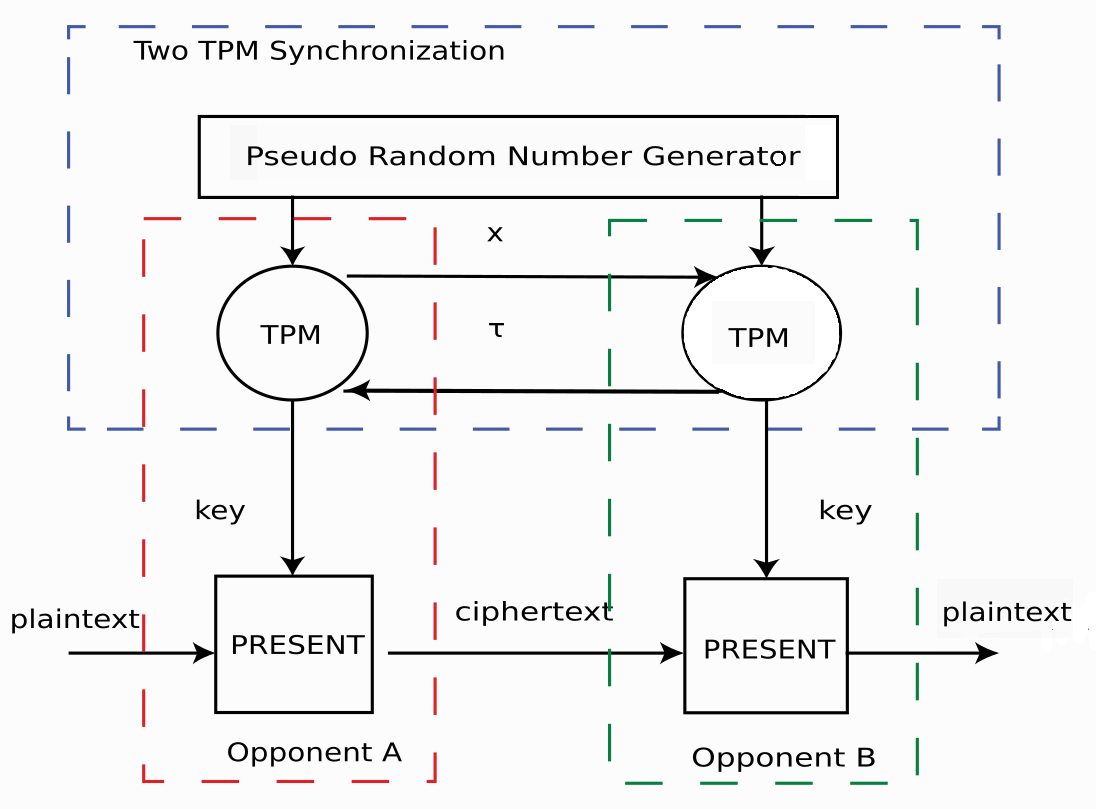Diagram 1 - Cryptography system diagram

The diagram describes two TPM synchronization and information exchage process. First, neural networks synchronize by mutual learning. Both of the communicating networks receive an identical input vector - x, generate an output bit - τ  and are trained on the corresponding bit of their partner. Then, plaintext encrypts with key and PRESENT cipher. Finally, the opponent gets ciphertext and decrypts it.Diagram 2 - PC-FPGA Diagram

#### 3. Intel FPGA Virtues in Your Project

FPGA solution suits for neural networks implementation as it preserves the parallel architecture of the neurons in a layer and offers flexibility in reconfiguration.

Additionally, PRESENT cipher used for encryption is an algorithm with a very low implementation complexity, especially in hardware. Being implemented in FPGA it provides compact, light on power solution.

One more significant advantage of this debug board is the  Intel FPGA OpenCL BSP. This allows to implement hardware accelerator to transfer it heavy calculations. The computation demanding tasks can be off-loaded from CPU to FPGA, resulting in significant system performance improvement.

OpenCL also includes an application programming interface (API) for the host to communicate with the hardware accelerator traditionally over PCI Express which provides high data transfer rate.

Moreover, this data transfer method between PC and debug board makes information exchange secure since unencrypted information sends only through PCI Express.

And last, but not least, supporting Intel FPGA OpenCL BSP can be used to design a system with high level programming language.

# TPM

As it has been already mentioned in “High-level Project Description” two synchronized TPM networks have identical synaptic weights. Based on TPMs weights common secret key can be generated and used in the symmetric-key algorithm.

All notations bellow marked with superscript A refers to the first network and others marked with B to the second one.

TPM is a particular multi-layer feedforward neural network structure employing the mutual learning concept for neural cryptography .  Each TPM consists of one output neuron, K hidden neurons and K*N input neurons.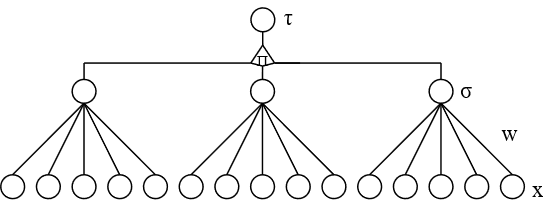Figure 1 - TPM structure (K=3)

According to the   input neurons xi,j and synaptic weights wi,j  can take values:

xi,j Î {-1,+1},  i Î {1,K} , j Î {1,N},

wi,jA/BÎ {-L,-L+1,…,L-1,L},

each of hidden layer bit siA/B defined as

siA=sign(wiA× xi), siB=sign(wiB× xi),

TPM output bit:

tA=s1As2As3A, tB=s1Bs2Bs3B

Learning process

As it is described in  the two output bits are used for the mutual training process. At each training step  two machines A and B receive identical random input vectors x1,x2,x3. The weights w1,w2,w initialize with the identical random values. The training algorithm is the following:

Only if the two output bits are identical, t = tB, the weights can be changed. In this case, only the hidden unit si  which is identical to t changes its weights using one of the following rules:

• Hebbian rule

wiA(t+1)= wiA(t-1)+tA/B xiΘ(siAtA/B)Θ(tAtB);

• Anti-hebbian rule

wiA(t+1)= wiA(t-1)-tA/B xiΘ(siAtA/B)Θ(tAtB);

• Random walk

wiA(t+1)= wiA(t-1)- xiΘ(siAtA/B)Θ(tAtB)

and the same for the network B. If this training step pushes any component wi,j out of the interval -L, ... , L the component is replaced by ±L, correspondingly.

# PRESENT Cipher

For the implemented symmetric cryptosystem PRESENT Cipher is chosen due to its low implementation complexity, especially in hardware. It has 64 bits block lengths and 80- or 128-bits key. Being  a substitution-permutation network (SP-network) it consists of 31 rounds.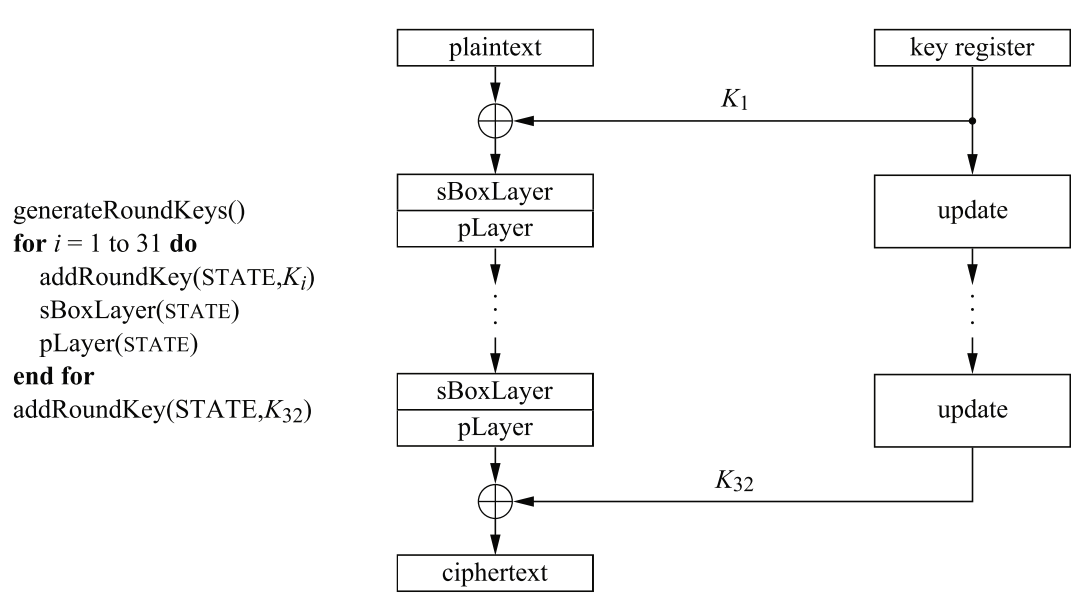Figure 2 - Internal structure and pseudocode of the block cipher PRESENT

Each round consists of 3 steps:

2. Non-linear substitution layer conversion
3. Bit-wise permutation layer conversion.

## Key schedule

The first subkey K1 is a direct copy of 64 bit of the user supplied key. For the following subkeys K2,...,K32 the key register K=k79k78...k0 is updated as follows:

1. [k79k78 ...k1k0]=[k18k17 ...k20k19]
2. [k79k78k77k76]= S[k79k78k77k76]
3. [k19k18k17k16k15]=[k19k18k17k16k15]⊕round_counter

## S-layer

The S-box used in PRESENT is a single 4-bit to 4-bit S-box which is applied 16 times in parallel. The action of the S-box is shown by the following table.

Table 1 - The PRESENT S-box in hexadecimal notation

x 0 1 2 3 4 5 6 7 8 9 A B C D E F
S[x] C 5 6 B 9 0 A D 3 E F 8 4 7 1 2

## P-layer

The bit permutation used in PRESENT is given by table:

 i 0 1 2 3 4 5 6 7 8 9 10 11 12 13 14 15 P(i) 0 16 32 48 1 17 33 49 2 18 34 50 3 19 35 51 i 16 17 18 19 20 21 22 23 24 25 26 27 28 29 30 31 P(i) 4 20 36 52 5 21 37 53 6 22 38 54 7 23 39 55 i 32 33 34 35 36 37 38 39 40 41 42 43 44 45 46 47 P(i) 8 24 40 56 9 25 41 57 10 26 42 58 11 27 43 59 i 48 49 50 51 52 53 54 55 56 57 58 59 60 61 62 63 P(i) 12 28 44 60 13 29 45 61 14 30 46 62 15 31 47 63

# Pseudo Random Number Generator

As reported above input TPM vectors and synaptic weights must be random numbers. In  Linear Feedback Shift Register(LFSR) is proposed for generating pseudo-random numbers.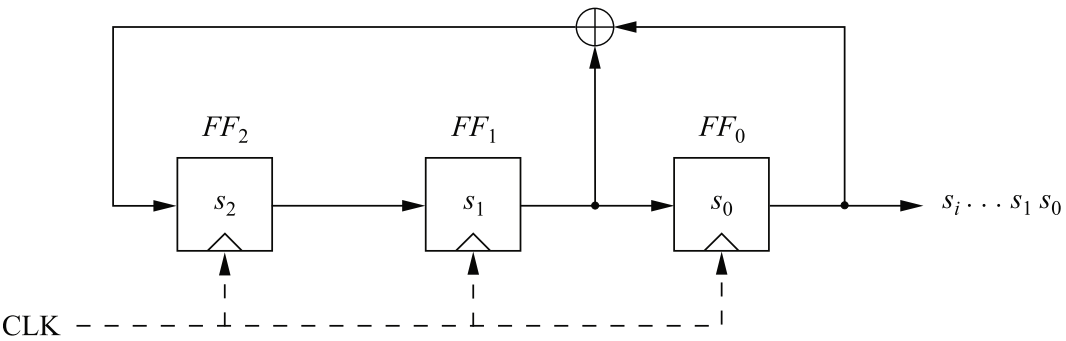Figure 3 - Linear feedback shift register of degree 3

LFSR includes shift register and feedback function. The described register consists of bits and its length is the number of these bits.

When an output bit is retrieved, all the other bits of the register are shifted to the right by one position. In this case, the new leftmost bit is determined by the function of the remaining bits.

It is advisable to use FPGA implementation because the linear feedback shift register can be represented as a series of Flip-Flops inside of an FPGA that are wired together as a shift register.

#### 5. Function Description

To develop a model of neurons and the neural network itself, it is necessary to develop their structure. As already mentioned in the 2nd chapter, the network consists of three layers of neurons: input, hidden and output.
To calculate the value of a hidden layer neuron, it is necessary to implement a module in which the sum of the products is calculated - w_i ^ A * x_i and the activation function module - sign (w_i ^ B * x_i).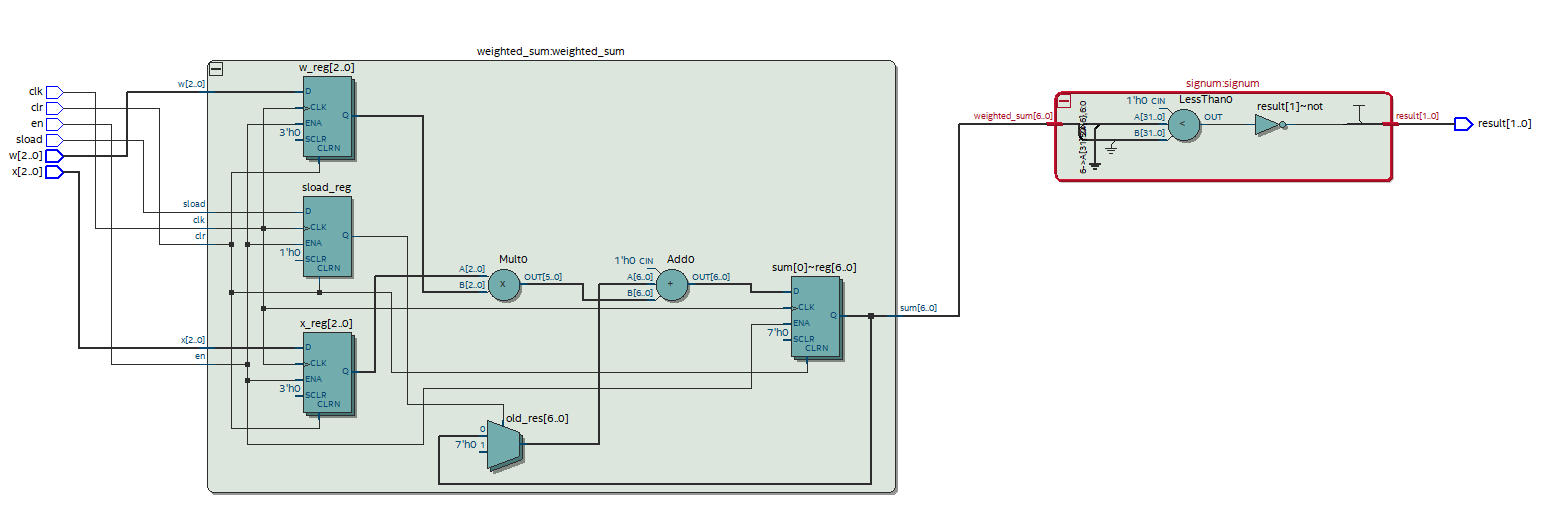Figure 4 - The structure of the neuron of the hidden layer

module hidden_layer_neuron - a module that implements a hidden layer neuron. module weighted_sum and module signum - modules that implement the multiplication of input data by their weight coefficients, followed by summation and activation function, respectively.
The weighted_sum module uses the property of input values x_ (i, j) ∈ {-1, + 1} and the input vectors are garnished with sequences of x_ (i, j) ∈ {0,1}. If x_ (i, j) = 0, the corresponding weight is subtracted from the previous result, x_ (i, j) = 1 - is added.

The value of the neuron of the output layer is calculated as the product of the output values of the neurons of the hidden layer t = σ_1 σ_2 σ_3.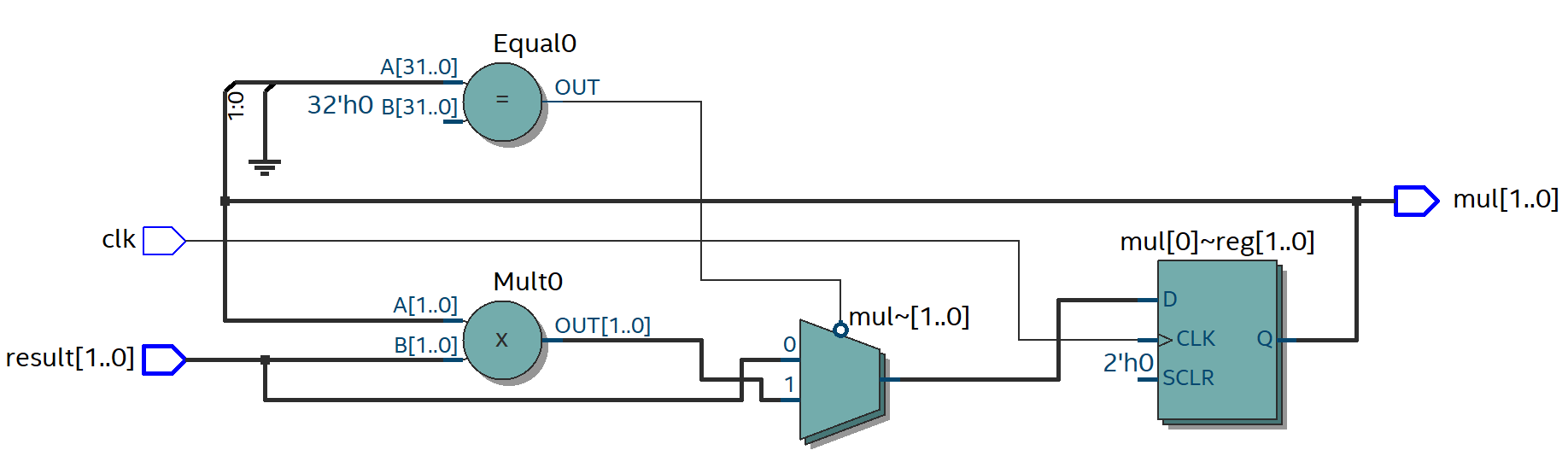Figure 5 - The structure of the neuron of the output layer

#### 6. Performance Parameters

Synthesis Results

The table shows the compilation results for a different number of neurons in the input layer. From the data presented, it can be concluded that TPM has sufficient scalability so that the generated key cannot be determined by enumeration according to the formula (2 ∙ L + 1) ^ KN.

Table 3 - the scalability of the neural network

 K × N × L 3 × 3 × 7 3 × 10 × 7 3 × 20 × 7 3 × 100 × 7 3 × 1000× 7 Logic utilization (in ALMs) 65 128 236 967 10384 Total registers 166 384 702 3194 30900 Total DSP Blocks 1 1 1 1 1

K is the number of neurons in the hidden layer, N is the number of neurons in the input layer.

Reliability analysis of a neurocryptographic system
Types of attacks and system reliability during key generation
For each attack, it is assumed that cryptanalyst E can eavesdrop on messages between A and B, but cannot change them.
Brute force method
This type of attack is characterized by checking all possible key options, that is, all weights w_ (i, j). For hidden K hidden neurons, K × N input neurons and weights -L, -L + 1, ..., L-1, L, the number of keys is (2 ∙ L + 1) ^ KN.
Let the cryptanalyst have the same TPM as the subscribers. At each synchronization step, three situations are possible:
t ^ A ≠ t ^ B - subscribers do not update weights.
t ^ A = t ^ B = t ^ E - A, B and E update the weights.
t ^ A = t ^ B ≠ t ^ E - A and B update the weights, but E cannot do this, so he learns more slowly than A and B synchronize.
Thus, the cryptanalyst can determine the key, but this will require a longer amount of time than synchronization.
Other attacks
In conventional cryptographic systems, to increase complexity, attacks increase the key length, in neurocryptography, instead of the length of the key itself, you can increase the synaptic length L. This increases the complexity of the attack exponentially, while the cost of decryption of subscribers increases polynomially. Thus, hacking such a system is NP challenging.
Types of attacks on the PRESENT cipher
Differential cryptanalysis
This cipher has the property that any 5 round differential characteristic involves at least 10 S box. Let the number of cipher rounds be 25, then at least 5 × 10 = 50 S-boxes will be involved. The maximum probability for one S box is 2 ^ (- 2), therefore, the probability of a 25-round characteristic will be no more than 2 ^ (- 100). Technologies exist that allow the cryptanalyst to remove rounds from the cipher in order to use a shorter characteristic. However, even if the attacker manages to remove six rounds from the cipher, the data necessary to use the remaining 25 round differential characteristic will exceed the permissible value. Thus, the security level is higher than required.
Linear cryptanalysis
The cipher has another property, namely that the maximum slope of the approximated straight line for 4 rounds does not exceed 1/2 ^ 7. Based on this statement, for 28 rounds, the maximum slope will be 2 ^ 6 × 1/2 / 7 ^ 2 = 2 ^ (- 43). Considering that hacking for round 31 requires an approximation for 28, you need 2 ^ 84 known source / ciphertext pairs, and this exceeds the size of a possible encryption test.

#### 7. Design Architecture

The figure shows a diagram of the developed system. A and B - two subscribers, each of which has a PC and FPGA. To exchange information in an encrypted form, subscribers must have a shared secret key and know the encryption algorithm. Keys are generated by neural networks during synchronization.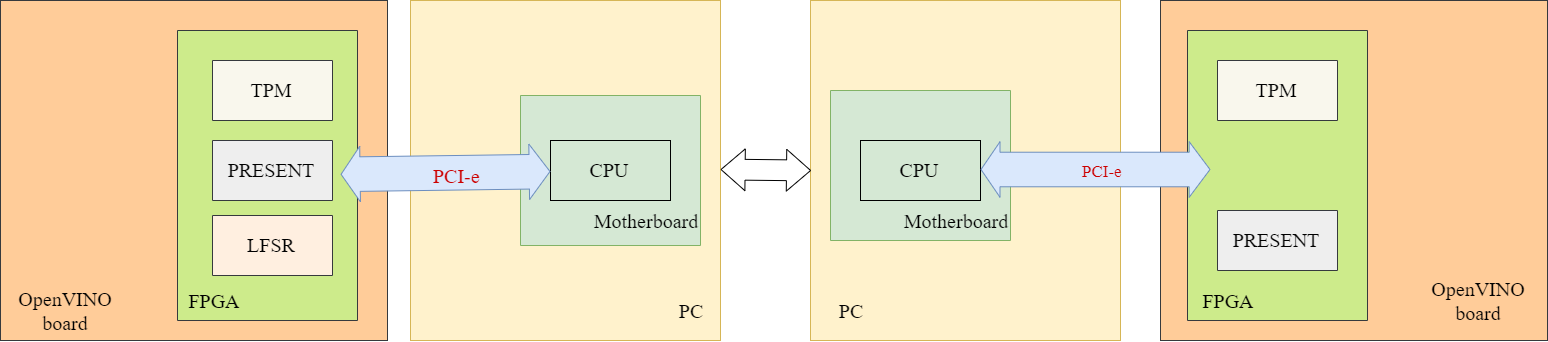The figure shows a diagram of a neurocryptographic system based on TPM networks and the PRESENT code. A and B are neural networks implemented on FPGAs A and B, respectively. According to the synchronization algorithm, the values of the input vectors and the initial values of the weight coefficients of each network are random numbers. Sequences of pseudo-random numbers are fed to the inputs by a pseudo-random number generator.After synchronization is completed, the weights of both networks are the same for any sets of the same input data and are updated after each information exchange session.
Let subscriber A want to send message B. The source text is generated on a PC belonging to A, then it is transmitted via UART to FPGA A. There, using the PRESENT cipher and key, the information is encrypted and transmitted to FPGA B (Figures 7, 8). Then the message is decrypted, after which it is sent to PC B.
Thus, the developed system remains of the three main modules:
- ANN TPM;
- a pseudo-random number generator;
- PRESENT cipher.

Conclusions

Due to the hardware implementation, the lightweight block cipher PRESENT was chosen, since according to the source [Paar C., Pelzl J. Understanding Cryptography. Berlin: Springer, 2010. 372 p.] It requires 2.5 times fewer logic elements than for AES.
After choosing an encryption algorithm, we studied the advantages of hardware implementation of neural networks on FPGAs, which consist in a higher speed of operation and less demanding resources.
An analysis of the theory of synchronization of tree-like parity machines shows that the synaptic weights of two mutually trained networks can be used as secret encryption keys. An important feature of this approach is that the same keys are not used twice.
To prototype TPM on the debug board, it was necessary to create an additional module to generate a sequence of pseudorandom numbers. For its implementation, a linear feedback shift register was selected.
The result of the work is an implemented system for the secure transmission of messages based on TPM networks with hardware implementation on the FPGA. This neurocryptographic system can be used, as in this work, to encrypt information transmitted from a PC, and as an IP core to accelerate encryption of transmitted information when developing systems on a chip.

References

1. Al-Jammas M., Z Othman K.M. Implementation of neural-cryptographic system using fpga // Artic. J. Eng. Sci. Technol. 2011. Vol. 44, № 6. P. 4111.
2. Volkmer M., Wallner S. Tree Parity Machine Rekeying Architectures. 2005. Vol. 54, № 4. P. 421–427.
3. Paar C., Pelzl J. Understanding Cryptography. Berlin: Springer, 2010. 372 p.
4. Omondi A.R., Rajapakse J.C. FPGA Implementations of Neural Networks / ed. Omondi A.R., Rajapakse J.C. Dordrecht: Springer, 2006.
5.  Bisalapur S.S., Jogdand R.M. Design of an Efficient Key Generation. 2011. Vol. 2, № 1. P. 60–69.
6. Hajduk Z. Reconfigurable FPGA implementation of neural networks // Neurocomputing. Elsevier B.V., 2018. Vol. 308. P. 227–234.
7. Allam A.M., Abbas H.M., El-Kharashi M.W. Security analysis of neural cryptography implementation // IEEE Pacific RIM Conference on Communications, Computers, and Signal Processing - Proceedings. IEEE, 2013. P. 195–199.
8. Wolfgang K., Kanter I. Neural cryptography / ed. Lipo Wan Jagath C Rappahe Kunihilo Cuku+htma. SmYoung Lee  and X.Y. 2002. P. 1351–1354.
9. Javurek M., Tur M. Synchronization of Two Tree Parity Machines // 2016 New Trends Signal Process. Armed Forces Academy of gen. M. R. Stefanik in Liptovsky Mikulas. Vol. 3. P. 1–4.
10. Volkmer M., Wallner S. Tree Parity Machine Rekeying Architectures // IEEE Trans. Comput. 2004. Vol. 54, № 4. P. 421–427.
11. Bogdanov A. et al. Cryptographic Hardware and Embedded Systems - CHES 2007 // PRESENT: An Ultra-Lightweight Block Cipher / ed. Paillier P., Verbauwhede I. Vienna, 2007. P. 447–466.

Alexander Samsonov
Good idea to use FPGA in calculation purposes
🕒 Jul 04, 2019 02:05 AM
igor Stolyarenko
Very deep knowledge
🕒 Jul 02, 2019 10:44 AM
Doreen Liu
By the way, we have released openvino 2019 R1 package for OSK, you can get the package from this link:
https://www.terasic.com.tw/cgi-bin/page/archive.pl?Language=English&CategoryNo=167&No=1159&PartNo=4
🕒 Jun 27, 2019 02:04 PM
Doreen Liu
An excellent proposal!
🕒 Jun 27, 2019 02:04 PM